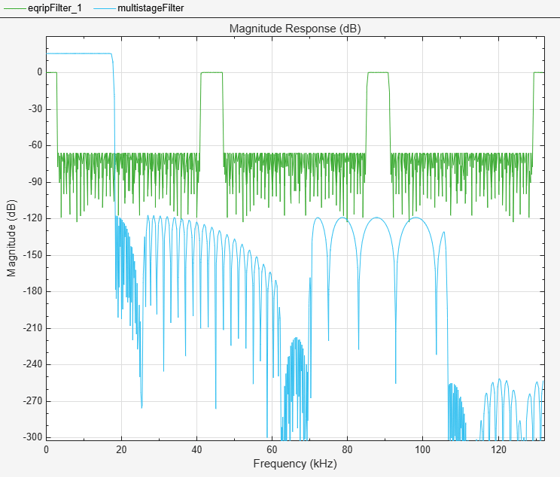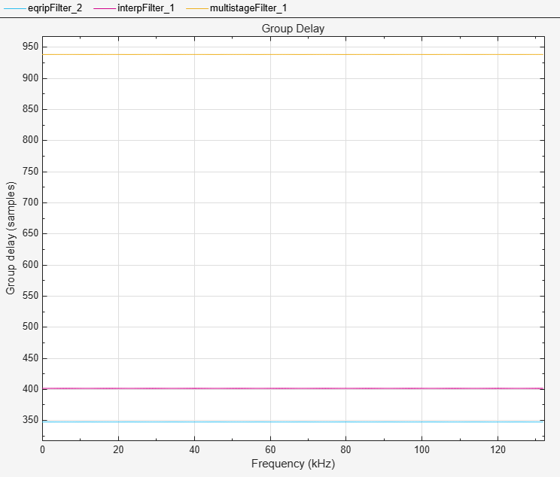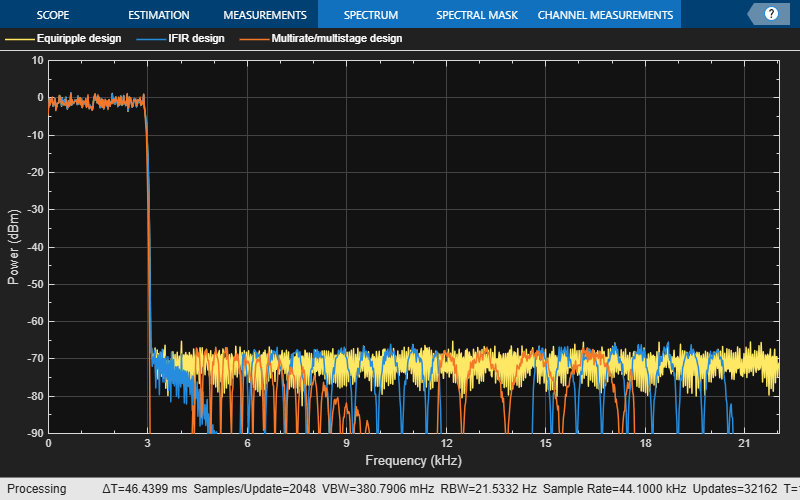# Efficient Narrow Transition-Band FIR Filter Design

This example shows how to design efficient FIR filters with very narrow transition-bands using multistage techniques. The techniques can be extended to the design of multirate filters. See Multistage Rate Conversion for an example of that.

### Design of a Lowpass Filter with Narrow Transition Bandwidth

One of the drawbacks of using FIR filters is that the filter order tends to grow inversely proportional to the transition bandwidth of the filter. Consider the following design specifications (where the ripples are given in linear units):

```Fpass = 2866.5; % Passband edge Fstop = 3087; % Stopband edge Apass = 0.0174; % Passband ripple, 0.0174 dB peak to peak Astop = 66.0206; % Stopband ripple, 66.0206 dB of minimum attenuation Fs = 44.1e3; lowpassSpec = fdesign.lowpass(Fpass,Fstop,Apass,Astop,Fs);```

A regular linear-phase equiripple design that meets the specs can be designed with:

```eqripFilter = design(lowpassSpec,'equiripple','SystemObject',true); cost(eqripFilter)```
```ans = struct with fields: NumCoefficients: 695 NumStates: 694 MultiplicationsPerInputSample: 695 AdditionsPerInputSample: 694 ```

The filter length required turns out to be 694 taps.

### Interpolated FIR (IFIR) Design

The IFIR design algorithm achieves an efficient design for the above specifications in the sense that it reduces the total number of multipliers required. To do this, the design problem is broken into two stages, a filter which is upsampled to achieve the stringent specifications without using many multipliers, and a filter which removes the images created when upsampling the previous filter.

`interpFilter = design(lowpassSpec,'ifir','SystemObject',true);`

Apparently we have made things worse. Instead of a single filter with 694 multipliers, we now have two filters with a total of 804 multipliers. However, close examination of the second stage shows that only about one multiplier in 5 is non-zero. The actual total number of multipliers has been reduced from 694 to 208.

`cost(interpFilter)`
```ans = struct with fields: NumCoefficients: 208 NumStates: 802 MultiplicationsPerInputSample: 208 AdditionsPerInputSample: 206 ```

Let's compare the responses of the two designs:

```fvt = fvtool(eqripFilter,interpFilter,'Color','White'); legend(fvt,'Equiripple design', 'IFIR design','Location','Best')```### Manually Controlling the Upsampling Factor

In the previous example, we automatically determined the upsampling factor used such that the total number of multipliers was minimized. It turned out that for the given specifications, the optimal upsampling factor was 5. However, if we examine the design options:

`opts = designopts(lowpassSpec,'ifir')`
```opts = struct with fields: FilterStructure: 'dffir' UpsamplingFactor: 'auto' JointOptimization: 0 SystemObject: 0 ```

we can see that we can control the upsampling factor. For example, if we wanted to upsample by 4 rather than 5:

```opts.UpsamplingFactor = 4; opts.SystemObject = true; upfilter = design(lowpassSpec,'ifir',opts); cost(upfilter)```
```ans = struct with fields: NumCoefficients: 217 NumStates: 767 MultiplicationsPerInputSample: 217 AdditionsPerInputSample: 215 ```

We would obtain a design that has a total of 217 non-zero multipliers.

### Using Joint Optimization

It is possible to design the two filters used in IFIR conjunctly. By doing so, we can save a significant number of multipliers at the expense of a longer design time (due to the nature of the algorithm, the design may also not converge altogether in some cases):

```opts.UpsamplingFactor = 'auto'; % Automatically determine the best factor opts.JointOptimization = true; ifirFilter = design(lowpassSpec,'ifir',opts); cost(ifirFilter)```
```ans = struct with fields: NumCoefficients: 172 NumStates: 726 MultiplicationsPerInputSample: 172 AdditionsPerInputSample: 170 ```

For this design, the best upsampling factor found was 6. The number of non-zero multipliers is now only 152

### Using Multirate/Multistage Techniques to Achieve Efficient Designs

For the designs discussed so far, single-rate techniques have been used. This means that the number of multiplications required per input sample (MPIS) is equal to the number of non-zero multipliers. For instance, the last design we showed requires 152 MPIS. The single-stage equiripple design we started with required 694 MPIS.

By using multirate/multistage techniques which combine decimation and interpolation we can also obtain efficient designs with a low number of MPIS. For decimators, the number of multiplications required per input sample (on average) is given by the number of multipliers divided by the decimation factor.

`multistageFilter = design(lowpassSpec,'multistage','SystemObject',true)`
```multistageFilter = dsp.FilterCascade with properties: Stage1: [1x1 dsp.FIRDecimator] Stage2: [1x1 dsp.FIRDecimator] Stage3: [1x1 dsp.FIRDecimator] Stage4: [1x1 dsp.FIRInterpolator] Stage5: [1x1 dsp.FIRInterpolator] Stage6: [1x1 dsp.FIRInterpolator] ```
`cost(multistageFilter)`
```ans = struct with fields: NumCoefficients: 396 NumStates: 352 MultiplicationsPerInputSample: 73 AdditionsPerInputSample: 70.8333 ```

The first stage has 21 multipliers, and a decimation factor of 3. Therefore, the number of MPIS is 7. The second stage has a length of 45 and a cumulative decimation factor of 6 (that is the decimation factor of this stage multiplied by the decimation factor of the first stage; this is because the input samples to this stage are already coming in at a rate 1/3 the rate of the input samples to the first stage). The average number of multiplications per input sample (reference to the input of the overall multirate/multistage filter) is thus 45/6=7.5. Finally, given that the third stage has a decimation factor of 1, the average number of multiplications per input for this stage is 130/6=21.667. The total number of average MPIS for the three decimators is 36.5.

For the interpolators, it turns out that the filters are identical to the decimators. Moreover, their computational cost is the same. Therefore the total number of MPIS for the entire multirate/multistage design is 73.

Now we compare the responses of the equiripple design and this one:

```fvt = fvtool(eqripFilter,multistageFilter,'Color','White', ... 'NormalizeMagnitudeto1','on'); legend(fvt,'Equiripple design', 'Multirate/multistage design', ... 'Location','NorthEast')```Notice that the stopband attenuation for the multistage design is about double that of the other designs. This is because it is necessary for the decimators to attenuate out of band components by the required 66 dB in order to avoid aliasing that would violate the required specifications. Similarly, the interpolators need to attenuate images by 66 dB in order to meet the specifications of this problem.

### Manually Controlling the Number of Stages

The multirate/multistage design that was obtained consisted of 6 stages. The number of stages is determined automatically by default. However, it is also possible to manually control the number of stages that result. For example:

```lp4stage=design(lowpassSpec,'multistage','NStages',4,'SystemObject',true); cost(lp4stage)```
```ans = struct with fields: NumCoefficients: 516 NumStates: 402 MultiplicationsPerInputSample: 86 AdditionsPerInputSample: 84.5000 ```

The average number of MPIS for this case is 86

### Group Delay

We can compute the group delay for each design. Notice that the multirate/multistage design introduces the most delay (this is the price to pay for a less computationally expensive design). The IFIR design introduces more delay than the single-stage equiripple design, but less so than the multirate/multistage design.

```fvt = fvtool(eqripFilter,interpFilter,multistageFilter,... 'Analysis','grpdelay','Color','White'); legend(fvt, 'Equiripple design','IFIR design',... 'Multirate/multistage design');```### Filtering a Signal

We now show by example that the IFIR and multistage/multirate design perform comparably to the single-stage equiripple design while requiring much less computation. In order to perform filtering for cascades, it is necessary to call generateFilteringCode(ifirFilter) and generateFilteringCode(multistageFilter). This has been done here already in order to create HelperIFIRFilter and HelperMultiFIRFilter.

```scope = dsp.SpectrumAnalyzer('SpectralAverages',50,'SampleRate',Fs,... 'PlotAsTwoSidedSpectrum',false,'YLimits', [-90 10],... 'ShowLegend',true','ChannelNames',{'Equiripple design',... 'IFIR design','Multirate/multistage design'}); tic, while toc < 20 % Run for 20 seconds x = randn(6000,1); % Filter using single stage FIR filter y1 = eqripFilter(x); % Filter using IFIR filter y2 = HelperIFIRFilter(x); % Filter multi-stage/multi-rate FIR filters y3 = HelperMultiFIRFilter(x); % Compare the output from both approaches scope([y1,y2,y3]) end```## SupportGet trial now